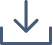### Randomization Techniques for Large-Scale Optimization

The interest in large-scale optimization methods has recently grown remarkably due to their application in diverse practical problems including machine learning, statistics and data science. Recent works have shown that the introduction of randomization techniques such as random shuffling in optimization algorithms would result in more efficient and accurate results. Yet, the mathematical justification of this is still lacking which servers as an obstruction on understanding the randomization techniques and developing new and efficient numerical methods. This project aims to introduce randomization techniques into the operator splitting algorithms for the more general nonconvex problems (such as the proximal gradient methods and the Douglas-Rachford algorithm). In particular, it will focus on how to use randomization techniques to improve current splitting algorithms for nonconvex optimization problems.#### Xiaoyu Li

The University of New South Wales

Xiaoyu Li is a fourth-year undergraduate student at the University of New South Wales majoring in mathematics and computer science. His research focus lies between applied mathematics and computer science, with interests in mathematical optimization and theoretical machine learning. He is working towards attaining a doctorate in mathematics and hopes to be qualified enough to become a mathematician someday, which would be a great honor.